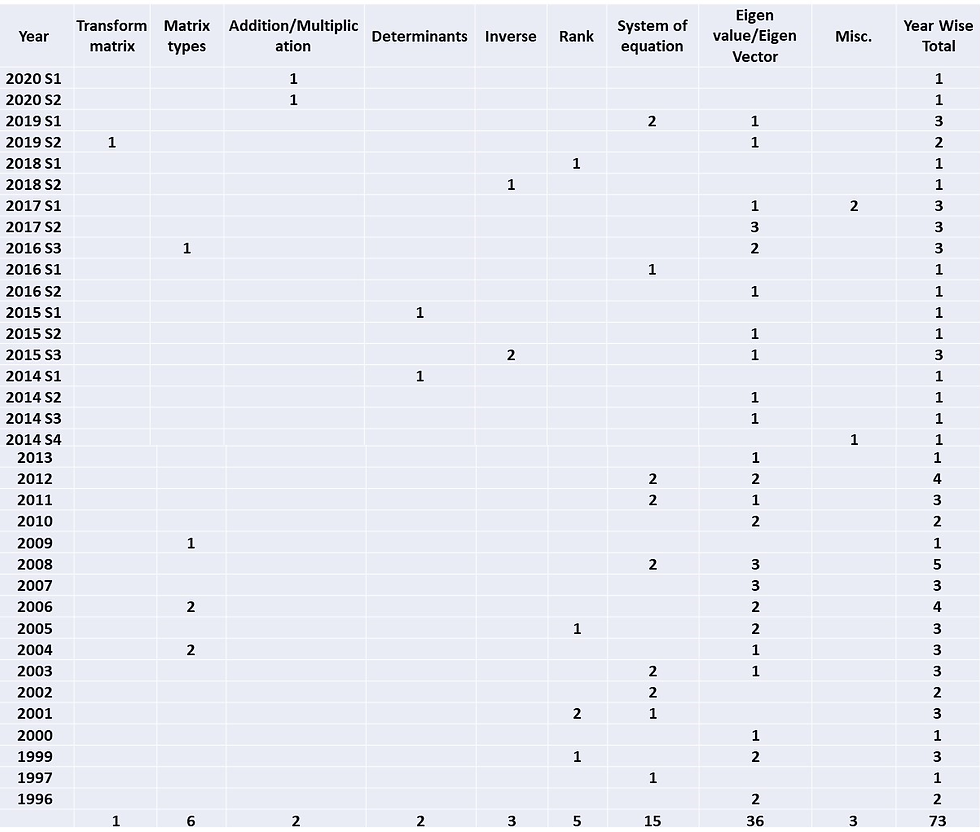top of pageSearch

# Matrix Algebra: Topic-wise distribution of previous years GATE questions

Topic-wise distribution of 25 years GATE questions asked from Matrix Algebra.

Engineering Mathematics is an important and easy to cover part of GATE paper. Around 15% marks in GATE Mechanical Engineering paper is allotted to engineering mathematics and with a little practice one can score 100% in this portion.

From matrix algebra, on an average 2 to 3 marks questions are asked every year. There are some topics like linear system of equations, eigenvalues and eigenvectors which are given more stress.

## Matrix Algebra: Topic-wise distribution of questions (on the basis of marks) asked in GATE ME paper 1996-2020## Year-wise distribution of questions from Matrix Algebra: GATE Mechanical Engineering 1996-2020Topic-wise detailed notes and previous years solved questions: